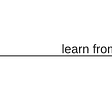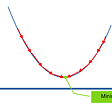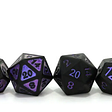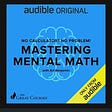# Algorithm

897. Increasing Order Search Tree

https://leetcode.com/problems/increasing-order-search-tree/

Given the `root` of a binary search tree, rearrange the tree in in-order so that the leftmost node in the tree is now the root of the tree, and every node has no left child and only one right child.

Example 1:

`Input: root = [5,3,6,2,4,null,8,1,null,null,null,7,9]Output: [1,null,2,null,3,null,4,null,5,null,6,null,7,null,8,null,9]`

Example 2:

`Input: root = [5,1,7]Output: [1,null,5,null,7]`

Constraints:

• The number of nodes in the given tree will be in the range `[1, 100]`.
• `0 <= Node.val <= 1000`

104. Maximum Depth of Binary Tree

Given the `root` of a binary tree, return its maximum depth.

A binary tree’s maximum depth is the number of nodes along the longest path from the root node down to the farthest leaf node.

Example 1:

`Input: root = [3,9,20,null,null,15,7]Output: 3`

Example 2:

`Input: root = [1,null,2]Output: 2`

Example 3:

`Input: root = []Output: 0`

Example 4:

`Input: root = Output: 1`

Constraints:

• The number of nodes in the tree is in the range `[0, 104]`.
• `-100 <= Node.val <= 100`

1137. N-th Tribonacci Number

https://leetcode.com/problems/n-th-tribonacci-number/

The Tribonacci sequence Tn is defined as follows:

T0 = 0, T1 = 1, T2 = 1, and Tn+3 = Tn + Tn+1 + Tn+2 for n >= 0.

Given `n`, return the value of Tn.

Example 1:

`Input: n = 4Output: 4Explanation:T_3 = 0 + 1 + 1 = 2T_4 = 1 + 1 + 2 = 4`

Example 2:

`Input: n = 25Output: 1389537`

Constraints:

• `0 <= n <= 37`
• The answer is guaranteed to fit within a 32-bit integer, ie. `answer <= 2^31 - 1`.

--

--

--

## More from Joo Hee Paige Kim

Consistency achieves everything https://github.com/paigekim29

Love podcasts or audiobooks? Learn on the go with our new app.

## Association vs Aggregation vs Composition## First Step: Creating a Whologram within the Hologram — Spontaneous Conversations 8## Sullivan’s Law## All About Betting Odds: What are they and how are they unfair?## No Calculator? No Problem! Mastering Mental Math by Art Benjamin## How to Use Math to Get Better at Anything## Joo Hee Paige Kim

Consistency achieves everything https://github.com/paigekim29

## What is a Dynamic Array?## Array Stepper Algorithm Problem